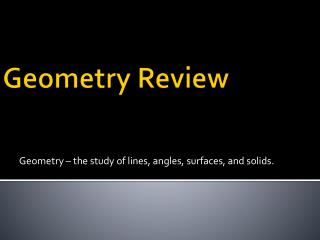DownloadDownload PresentationGeometry Review

# Geometry Review

Download Presentation## Geometry Review

- - - - - - - - - - - - - - - - - - - - - - - - - - - E N D - - - - - - - - - - - - - - - - - - - - - - - - - - -
##### Presentation Transcript

1. Geometry – the study of lines, angles, surfaces, and solids. Geometry Review

2. Types of Angles • Acute – an angle less than 90°. • Right – a 90° angle. • Obtuse – an angle between 90° and 180°. • Straight – a 180° angle. • Reflex – an angle between 180° and 360°. • Perigon – a 360° angle.

3. Other Types of Angles • Supplementary – angles that add up to 180°. • Complementary – angles that add up to 90°. • Opposite – when 2 lines cross the angles across from each other are always equal.

4. Types of Triangles • Equilateral – a triangle with 3 equal sides • Isosceles – a triangle with 2 equal sides • Scalene – a triangle with no equal sides • Right angled – a triangle with one 90° angle. • Obtuse Triangle • Acute Triangle • Right angled Isosceles Triangle

5. Triangle Property – the sum of the interior angles of any triangle add up to 180°. • Parallel lines – run beside each other and never meet. • Perpendicular lines – intersect at 90°.

6. Patterns with Parallel Lines • Alternate angles of a Z pattern are equal. • Corresponding angles of an F pattern are equal. • Co-interior angles of a C pattern are supplementary.

7. The Pythagorean Theorem

8. Right Triangle • Two shorter sides are called the legs • a & b • The hypotenuse is the side opposite the right angle (longest side) • c

9. The Pythagorean Theorem • The square of the hypotenuse is equal to the sum of the squares of the legs. • Used to find the length of any side of the triangle if given the other two sides.

10. Example: • Norma is planning to wash the windows on her house. She rests a 3m long ladder against the side of the house. The foot of the ladder is 1m from the house. How high up the house will the ladder reach? Round your answer to the nearest hundredth of a metre.